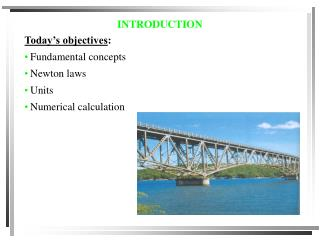# INTRODUCTION - PowerPoint PPT PresentationDownload PresentationINTRODUCTION

INTRODUCTIONDownload Presentation## INTRODUCTION

- - - - - - - - - - - - - - - - - - - - - - - - - - - E N D - - - - - - - - - - - - - - - - - - - - - - - - - - -
##### Presentation Transcript

1. INTRODUCTION • Today’s objectives: • Fundamental concepts • Newton laws • Units • Numerical calculation

2. WHAT IS MECHANICS?? • Study of what happens to a particle/body when FORCES are applied to it. • Either the body or the forces could be large or small.

3. WHERE IS MECHANICS?? Physics Electromagnetism Mechanics Optics Thermodynamics

4. WHO STARTED IT ALL! He managed, for the first time, to explain the relation between different physical quantities using: • A Mathematical language (calculus) • Experimental laws such as: Sir Isaac Newton 1643 - 1727 See his biography at: http://www-groups.dcs.st-and.ac.uk/~history/Mathematicians/Newton.html

5. DYNAMICS • Dynamics is the study of the motion of the objects: • Kinematicsis the study of the geometry of the motion or how position, velocity and acceleration of a moving object are related. (First part of this course) • Kinetics deals with how motion is caused by the applied forces. (Second part of this course)

6. TWO IMPORTANT IDEALIZATION • Particle vs. Rigid Body • In Rigid body, we should consider both mass and size • Rigid body has both translational and rotational motion • In Particle, mass is considered but “size” is neglected • Particle has no rotational motion • Rule of thumb: a finite body can be considered as a particle as long as the rotation of the body is not important in the resulting motion

7. TWO IMPORTANT IDEALIZATION • Concentrated force vs. Distributed force • The hydrostatic force of the water behind a dam is distributed over the surface • The cable force is a concentrated force or point force since it is applied to a point on the crate. • In dynamics of particle we usually deal with point force or concentrated forces

8. m QUANTITIES • Basic Quantities: • Length, • Time • Mass  velocity, acceleration • Force (not independent from the first three) F=ma

9. UNIT SYSTEMS • Note: • Unit is a part of a physical quantity. Don’t forget to report the units in your calculations and final answers • It is worth memorizing some simple unit conversion factors: • 1 ft = 0.3048 m, 1 lb = 4.4482 N, 1 slug = 14.5938 kg

10. NUMERICAL CALCULATIONS (review) • Must have dimensional “homogeneity.” Dimensions have to be the same on both sides of the equal sign, (e.g. distance = speed  time.) • Use an appropriate number of significant figures (3 for • answers, at least 4 for intermediate calculations). • Be consistent when rounding off. • - greater than 5, round up (3528  3530) • - smaller than 5, round down (0.03521  0.0352) • - equal to 5 and zeros in the following digits, then round to the closest even digit (2415  2420 or 2425  2420.

11. SAMPLE CONCEPT QUIZ 1. Give the most appropriate reason for using three significant figures in reporting results of typical engineering calculations. A) Historically, slide rulers could not handle more than three significant figures. B)Three significant figures gives better than one-percent accuracy. C) Telephone systems designed by engineers have area codes consisting of three figures. D) Most of the original data used in engineering calculations do not have accuracy better than one percent